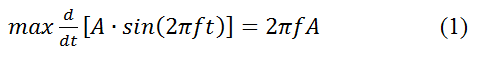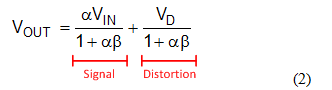Other Parts Discussed in Post: OPA1688, OPA1622

This post is co-authored by Bharath Vasan.

Pop quiz! Take a look at the total harmonic distortion and noise (THD+N) curve in Figure 1. It’s for an OPA1688, a 36V, 10MHz audio operational amplifier (op amp), delivering a 1VRMS sine wave to several common headphone impedances. The distortion at high frequency is rising and is worse for low impedances.

Why?

Choose the correct response:

(a)    The slew rate of the op amp is insufficient to reproduce the signal.
(b)   The op amp’s loop gain is decreasing.
(c)    The op amp’s output stage distorts more with low impedance loads.
(d)   Both B and C.Figure 1: THD+N vs. frequency curve for an OPA1688 with various loads

For those of you who answered “A,” slew rate is a measurement of how quickly an amplifier can change its output voltage in response to the input signal. If the amplifier’s slew rate is less than the maximum rate of change of the input signal, the amplifier will distort the signal. In the case of a sinusoid, the maximum rate of change depends on the frequency and amplitude (Equation 1):Insert some numbers from the quiz question into Equation 1 (1VRMS = 1.414Vp, 20kHz), and you can calculate the minimum amplifier slew rate necessary to reproduce the 1VRMS signal:This is a modest slew-rate requirement, and the OPA1688’s specification of 8V/μs is more than capable of preventing distortion from slew-rate limitations. Sorry, the answer isn’t A.

Now let’s address answers B and C: amplifier loop gain and output stage distortion. Consider the control system diagram in Figure 2, representing a basic op amp. The input stage of the op amp subtracts the feedback signal from the input voltage (VIN) and amplifies the difference by the open-loop gain: α. β is the feedback factor and represents the portion of the output voltage (VOUT) that is fed back to the input. The output stage of the op amp is the circuitry that delivers current to the load, represented by a simple unity-gain buffer. Figure 2 also includes a distortion source, VD, summed with the signal inside the control loop.Figure 2: A control-loop diagram of an op amp that includes an internal source of distortion

The output signal of this system consists of two terms: the input signal (now amplified) and some remaining distortion (Equation 2):The amount of distortion in the output signal is determined by:

• The amount of loop gain (the product of α and β).
• The amount of intrinsic distortion produced by the amplifier (the magnitude of VD).

In Figure 1, the loop gain of the amplifier is decreasing at a high frequency, which causes the corresponding rise in distortion (answer B). Now you know why the distortion would increase at high frequencies. This also explains why TI chose to maximize the open-loop gain of the OPA1622 at audio frequencies, as we described in part 2 of this blog series.

But answer B doesn’t explain why the distortion would be worse for low impedance loads. To understand this, we need to look at the gain of the output stage, which we assumed to be 1 in Figure 2. We’ll tackle that in a post next week, where we’ll show how the load impedance affects the gain of the output stage and compare the performance of the OPA1622 to the OPA1688 in the same test conditions.